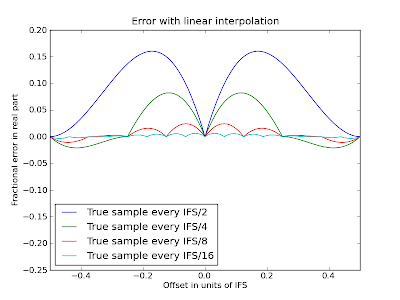### Coherent harmonic summingAs I alluded to in a previous post, you can (and it is sometimes useful to) take a giant FFT of a time series, extract a series of harmonically-related coefficients, and with an inverse FFT, produce a "pulse profile" showing the data "folded" at the period of the fundamental. This is interesting in part because you can use the peak height to gauge the strength of the signal, taking advantage of the relative phases of the harmonics. My question today is, when you're extracting those coefficients, how accurate do you need to be?

If all you want is the power, then a first approximation would be to simply choose the nearest Fourier frequency. This, after all, is where your FFT naturally measures the coefficient. I'll call these "independent Fourier frequencies", and the spacing between them the "independent Fourier spacing" (IFS). It turns out that if you have a frequency exactly halfway between two independent Fourier frequencies, you lose a substantial amount of sensitivity: a nearly 36% loss of signal-to-noise. You can cut this back to something like 7.4% using an ultra-simple interpolation scheme called "interbinning".

What about coherent reconstruction? Well, now we need not just the amplitude but the phase. The amplitude is relatively easy to get close to since it's at a maximum at the point of interest, so that the first derivative is zero and you have little dependence on the frequency. The phase does not have an extremum at the correct frequency, so it may well be varying rapidly. In fact, for a signal that is present uniformly throughout the observation, the phase changes by pi units per IFS. So if we use a spacing of IFS/2, which would have been adequate for the power, our phase will be wrong by as much as forty-five degrees.

What we care about, though, is not the phase exactly, but the real part. More, there are really two questions here: how well do we need to interpolate the FFT to get reasonably accurate Fourier coefficients, and how closely must we space our inverse FFTs?

First the easy question: how well do we need to interpolate to get decent Fourier coefficients. There are techniques for doing really good-quality interpolation in FFTs - you can use sinc interpolation (based on the 32 nearest samples), or you can just pad the time series before taking your giant FFT. But this is somewhat expensive. Since flops are free as long as they only access memory you've recently read anyway, to speed things up you can always linearly interpolate between more-carefully interpolated samples. So here's a plot of the error introduced by that linear interpolation:So if you do proper Fourier interpolation to a spacing of IFS/8, and linear interpolation beyond that, then your measured amplitude will be off by less than about 3%. Using IFS/4 still leaves the worst-case error at about 7%, about the same as interbinning.

Now for the tougher question: how far off can our estimate of the frequency be when we reconstruct our profile? This is crucial, since it determines how many inverse FFTs we need to do, and these are probably the most expensive part of the calculation. We can safely think of this in the time domain: we have a narrowly-peaked pulsar, and we're folding the incoming data at a slightly wrong frequency. How much does this lower the peak amplitude?

Well, if the frequency is wrong, over the course of the observation the pulsar's peak will drift in phase. The average profile will then be smeared out by the amount of drift. Exactly how much it will lose in peak height depends on the pulse shape. An error in frequency of one IFS will result in one full cycle of drift (in fact this is what defines an IFS). So if we are going up to the nth harmonic, then an error in (fundamental) frequency of IFS/n results in one full turn of drift for that harmonic, and we might as well not have bothered with that harmonic. But focusing on an individual harmonic can answer the question.

The loss in amplitude of a harmonic whose frequency is off by x is given by the sinc function; if we approximate the sinc function with its quadratic Taylor polynomial, we get a loss equal to x2π2/6, where x is the frequency error in IFS. Now, if we suppose that the profile is effectively a delta function, so that its n coefficients are all 1/n, then the total error is the sum over m of m2x2π2/6n or x2n(n+1)(2n+1)π2/36n.

What this works out to, once you clear up the messy math, is that if you sample the top harmonic at IFS/2, you lose about 14%; if you sample at IFS/4 you lose about 3.6%, and if the odd number doesn't make you queasy and you sample at IFS/3 you lose about 6.3%. (It turns out the number of harmonics is nearly irrelevant.)

So, in short, if you interpolate the FFT to IFS/8 and linearly interpolate beyond that, and you take an FFT every time you advance the top harmonic by its IFS/4. you'll lose no more than about 5% sensitivity at worst. If you cut your number of FFTs in half (which probably cuts your runtime in half), you lose at worst maybe 20% of your sensitivity, but you can probably make it up by setting a threshold 20% lower, then "tuning up" each candidate to find the best period.